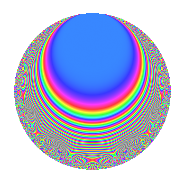# Properties

 Label 670.2.aLevel 670 Weight 2 Character orbit a Rep. character $$\chi_{670}(1,\cdot)$$ Character field $$\Q$$ Dimension 21 Newforms 10 Sturm bound 204 Trace bound 3

# Learn more about

## Defining parameters

 Level: $$N$$ = $$670 = 2 \cdot 5 \cdot 67$$ Weight: $$k$$ = $$2$$ Character orbit: $$[\chi]$$ = 670.a (trivial) Character field: $$\Q$$ Newforms: $$10$$ Sturm bound: $$204$$ Trace bound: $$3$$ Distinguishing $$T_p$$: $$3$$, $$7$$

## Dimensions

The following table gives the dimensions of various subspaces of $$M_{2}(\Gamma_0(670))$$.

Total New Old
Modular forms 106 21 85
Cusp forms 99 21 78
Eisenstein series 7 0 7

The following table gives the dimensions of the cuspidal new subspaces with specified eigenvalues for the Atkin-Lehner operators and the Fricke involution.

$$2$$$$5$$$$67$$FrickeDim.
$$+$$$$+$$$$+$$$$+$$$$2$$
$$+$$$$+$$$$-$$$$-$$$$4$$
$$+$$$$-$$$$+$$$$-$$$$3$$
$$+$$$$-$$$$-$$$$+$$$$2$$
$$-$$$$+$$$$+$$$$-$$$$3$$
$$-$$$$+$$$$-$$$$+$$$$2$$
$$-$$$$-$$$$+$$$$+$$$$2$$
$$-$$$$-$$$$-$$$$-$$$$3$$
Plus space$$+$$$$8$$
Minus space$$-$$$$13$$

## Trace form

 $$21q$$ $$\mathstrut -\mathstrut q^{2}$$ $$\mathstrut -\mathstrut 4q^{3}$$ $$\mathstrut +\mathstrut 21q^{4}$$ $$\mathstrut -\mathstrut q^{5}$$ $$\mathstrut -\mathstrut 4q^{6}$$ $$\mathstrut -\mathstrut 8q^{7}$$ $$\mathstrut -\mathstrut q^{8}$$ $$\mathstrut +\mathstrut 17q^{9}$$ $$\mathstrut +\mathstrut O(q^{10})$$ $$21q$$ $$\mathstrut -\mathstrut q^{2}$$ $$\mathstrut -\mathstrut 4q^{3}$$ $$\mathstrut +\mathstrut 21q^{4}$$ $$\mathstrut -\mathstrut q^{5}$$ $$\mathstrut -\mathstrut 4q^{6}$$ $$\mathstrut -\mathstrut 8q^{7}$$ $$\mathstrut -\mathstrut q^{8}$$ $$\mathstrut +\mathstrut 17q^{9}$$ $$\mathstrut +\mathstrut q^{10}$$ $$\mathstrut -\mathstrut 4q^{12}$$ $$\mathstrut -\mathstrut 14q^{13}$$ $$\mathstrut -\mathstrut 4q^{14}$$ $$\mathstrut +\mathstrut 4q^{15}$$ $$\mathstrut +\mathstrut 21q^{16}$$ $$\mathstrut -\mathstrut 6q^{17}$$ $$\mathstrut +\mathstrut 3q^{18}$$ $$\mathstrut -\mathstrut 12q^{19}$$ $$\mathstrut -\mathstrut q^{20}$$ $$\mathstrut +\mathstrut 16q^{21}$$ $$\mathstrut -\mathstrut 8q^{22}$$ $$\mathstrut +\mathstrut 12q^{23}$$ $$\mathstrut -\mathstrut 4q^{24}$$ $$\mathstrut +\mathstrut 21q^{25}$$ $$\mathstrut +\mathstrut 14q^{26}$$ $$\mathstrut +\mathstrut 8q^{27}$$ $$\mathstrut -\mathstrut 8q^{28}$$ $$\mathstrut +\mathstrut 6q^{29}$$ $$\mathstrut -\mathstrut 4q^{30}$$ $$\mathstrut -\mathstrut 8q^{31}$$ $$\mathstrut -\mathstrut q^{32}$$ $$\mathstrut +\mathstrut 40q^{33}$$ $$\mathstrut -\mathstrut 2q^{34}$$ $$\mathstrut +\mathstrut 17q^{36}$$ $$\mathstrut +\mathstrut 2q^{37}$$ $$\mathstrut -\mathstrut 4q^{38}$$ $$\mathstrut +\mathstrut q^{40}$$ $$\mathstrut +\mathstrut 18q^{41}$$ $$\mathstrut -\mathstrut 20q^{43}$$ $$\mathstrut +\mathstrut 3q^{45}$$ $$\mathstrut -\mathstrut 12q^{46}$$ $$\mathstrut -\mathstrut 4q^{47}$$ $$\mathstrut -\mathstrut 4q^{48}$$ $$\mathstrut -\mathstrut 15q^{49}$$ $$\mathstrut -\mathstrut q^{50}$$ $$\mathstrut -\mathstrut 14q^{52}$$ $$\mathstrut -\mathstrut 22q^{53}$$ $$\mathstrut -\mathstrut 40q^{54}$$ $$\mathstrut -\mathstrut 12q^{55}$$ $$\mathstrut -\mathstrut 4q^{56}$$ $$\mathstrut -\mathstrut 16q^{57}$$ $$\mathstrut -\mathstrut 6q^{58}$$ $$\mathstrut +\mathstrut 4q^{59}$$ $$\mathstrut +\mathstrut 4q^{60}$$ $$\mathstrut -\mathstrut 38q^{61}$$ $$\mathstrut -\mathstrut 8q^{62}$$ $$\mathstrut -\mathstrut 8q^{63}$$ $$\mathstrut +\mathstrut 21q^{64}$$ $$\mathstrut +\mathstrut 6q^{65}$$ $$\mathstrut +\mathstrut 16q^{66}$$ $$\mathstrut +\mathstrut q^{67}$$ $$\mathstrut -\mathstrut 6q^{68}$$ $$\mathstrut +\mathstrut 32q^{69}$$ $$\mathstrut -\mathstrut 8q^{70}$$ $$\mathstrut +\mathstrut 12q^{71}$$ $$\mathstrut +\mathstrut 3q^{72}$$ $$\mathstrut -\mathstrut 14q^{73}$$ $$\mathstrut -\mathstrut 2q^{74}$$ $$\mathstrut -\mathstrut 4q^{75}$$ $$\mathstrut -\mathstrut 12q^{76}$$ $$\mathstrut +\mathstrut 64q^{77}$$ $$\mathstrut +\mathstrut 8q^{78}$$ $$\mathstrut -\mathstrut 32q^{79}$$ $$\mathstrut -\mathstrut q^{80}$$ $$\mathstrut +\mathstrut 45q^{81}$$ $$\mathstrut -\mathstrut 2q^{82}$$ $$\mathstrut +\mathstrut 60q^{83}$$ $$\mathstrut +\mathstrut 16q^{84}$$ $$\mathstrut +\mathstrut 6q^{85}$$ $$\mathstrut +\mathstrut 20q^{86}$$ $$\mathstrut +\mathstrut 24q^{87}$$ $$\mathstrut -\mathstrut 8q^{88}$$ $$\mathstrut -\mathstrut 34q^{89}$$ $$\mathstrut +\mathstrut 13q^{90}$$ $$\mathstrut -\mathstrut 24q^{91}$$ $$\mathstrut +\mathstrut 12q^{92}$$ $$\mathstrut -\mathstrut 16q^{93}$$ $$\mathstrut -\mathstrut 12q^{94}$$ $$\mathstrut -\mathstrut 4q^{95}$$ $$\mathstrut -\mathstrut 4q^{96}$$ $$\mathstrut -\mathstrut 26q^{97}$$ $$\mathstrut +\mathstrut 23q^{98}$$ $$\mathstrut +\mathstrut 24q^{99}$$ $$\mathstrut +\mathstrut O(q^{100})$$

## Decomposition of $$S_{2}^{\mathrm{new}}(\Gamma_0(670))$$ into irreducible Hecke orbits

Label Dim. $$A$$ Field CM Traces A-L signs $q$-expansion
$$a_2$$ $$a_3$$ $$a_5$$ $$a_7$$ 2 5 67
670.2.a.a $$1$$ $$5.350$$ $$\Q$$ None $$-1$$ $$-2$$ $$1$$ $$-1$$ $$+$$ $$-$$ $$-$$ $$q-q^{2}-2q^{3}+q^{4}+q^{5}+2q^{6}-q^{7}+\cdots$$
670.2.a.b $$1$$ $$5.350$$ $$\Q$$ None $$-1$$ $$0$$ $$1$$ $$1$$ $$+$$ $$-$$ $$-$$ $$q-q^{2}+q^{4}+q^{5}+q^{7}-q^{8}-3q^{9}+\cdots$$
670.2.a.c $$1$$ $$5.350$$ $$\Q$$ None $$1$$ $$-2$$ $$-1$$ $$1$$ $$-$$ $$+$$ $$-$$ $$q+q^{2}-2q^{3}+q^{4}-q^{5}-2q^{6}+q^{7}+\cdots$$
670.2.a.d $$1$$ $$5.350$$ $$\Q$$ None $$1$$ $$0$$ $$-1$$ $$-5$$ $$-$$ $$+$$ $$-$$ $$q+q^{2}+q^{4}-q^{5}-5q^{7}+q^{8}-3q^{9}+\cdots$$
670.2.a.e $$2$$ $$5.350$$ $$\Q(\sqrt{2})$$ None $$-2$$ $$0$$ $$-2$$ $$2$$ $$+$$ $$+$$ $$+$$ $$q-q^{2}+\beta q^{3}+q^{4}-q^{5}-\beta q^{6}+(1+\cdots)q^{7}+\cdots$$
670.2.a.f $$2$$ $$5.350$$ $$\Q(\sqrt{2})$$ None $$2$$ $$-4$$ $$2$$ $$-6$$ $$-$$ $$-$$ $$+$$ $$q+q^{2}+(-2+\beta )q^{3}+q^{4}+q^{5}+(-2+\cdots)q^{6}+\cdots$$
670.2.a.g $$3$$ $$5.350$$ 3.3.148.1 None $$-3$$ $$4$$ $$3$$ $$1$$ $$+$$ $$-$$ $$+$$ $$q-q^{2}+(1+\beta _{1})q^{3}+q^{4}+q^{5}+(-1+\cdots)q^{6}+\cdots$$
670.2.a.h $$3$$ $$5.350$$ 3.3.756.1 None $$3$$ $$0$$ $$-3$$ $$3$$ $$-$$ $$+$$ $$+$$ $$q+q^{2}-\beta _{1}q^{3}+q^{4}-q^{5}-\beta _{1}q^{6}+\cdots$$
670.2.a.i $$3$$ $$5.350$$ 3.3.404.1 None $$3$$ $$2$$ $$3$$ $$1$$ $$-$$ $$-$$ $$-$$ $$q+q^{2}+(1-\beta _{1})q^{3}+q^{4}+q^{5}+(1-\beta _{1}+\cdots)q^{6}+\cdots$$
670.2.a.j $$4$$ $$5.350$$ 4.4.15188.1 None $$-4$$ $$-2$$ $$-4$$ $$-5$$ $$+$$ $$+$$ $$-$$ $$q-q^{2}+(-1-\beta _{2})q^{3}+q^{4}-q^{5}+(1+\cdots)q^{6}+\cdots$$

## Decomposition of $$S_{2}^{\mathrm{old}}(\Gamma_0(670))$$ into lower level spaces

$$S_{2}^{\mathrm{old}}(\Gamma_0(670)) \cong$$ $$S_{2}^{\mathrm{new}}(\Gamma_0(67))$$$$^{\oplus 4}$$$$\oplus$$$$S_{2}^{\mathrm{new}}(\Gamma_0(134))$$$$^{\oplus 2}$$$$\oplus$$$$S_{2}^{\mathrm{new}}(\Gamma_0(335))$$$$^{\oplus 2}$$